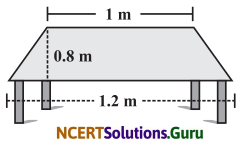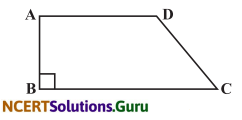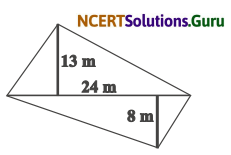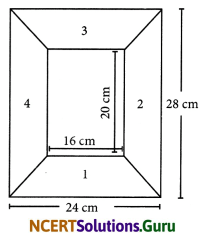# NCERT Solutions for Class 8 Maths Chapter 11 Mensuration Ex 11.2

These NCERT Solutions for Class 8 Maths Chapter 11 Mensuration Ex 11.2 Questions and Answers are prepared by our highly skilled subject experts.

## NCERT Solutions for Class 8 Maths Chapter 11 Mensuration Exercise 11.2

Question 1.
The shape of the top surface of a table is a trapezium. Find its area if its parallel sides are 1 m and 1.2 m and perpendicular distance between them is 0.8 m.Here, = a = 1.2m b = 1m and h = 0.8m
Area of a trapezium = $$\frac{1}{2}$$ × h × (a + b)
= $$\frac{1}{2}$$ × 0.8 × (1.2 + 1)m2 = 0.4 × 2.2 = 0.88 m2

Question 2.
The area of trapezium is 34 cm2 and the length of one of the parallel sides is 10 cm and its height is 4 cm. Find the length of the other parallel side.
Let the length of the other parallel side be ‘X’, height of a trapezium = 4 m. Length of one parallel side = 10 cmArea of a trapezium = 34 cm2
$$\frac{1}{2}$$ × h × (a + b) = 34
$$\frac{1}{2}$$ × 4 × (10 + x) = 34
2 (10 + x) = 34
20 + 2x = 34
2x = 34 – 20
2x = 14
x = $$\frac{14}{2}$$ = 7 cm
Hence, length of the other side = 7 cmQuestion 3.
Length of the fence of a trapezium shaped field ABCD is 120 m. If BC = 48 m, CD = 17m and AD = 40 m, find the area of this field. Side AB is perpendicular to the parallel sides AD and BC.
Given BC = 48m,CD = 17mandAD = 40m
Perimeter of a trapezium = 120 m
AB + BC + CD + DA = 120
AB + 48 + 17 + 40 = 120
AB + 105 = 120
AB = 120 – 105 = 15 m
AB is the height of the trapezium.
Area of a trapezium = $$\frac{1}{2}$$ × h × (a + b)
= $$\frac{1}{2}$$ × 15 × (48 + 40) m2
= $$\frac{1}{2}$$ × 15 × 88 m2 = 15 × 44 m2 = 660 m2
Area of the given trapezium = 660 m2

Question 4.
The diagonal of quadrilateral shaped field is 24 m and the perpendiculars dropped on it from the remaining opposite vertices are 8 m and 13 m. Find the area of the field.In the given quadrilateral, d = 24 m, h1 = 13 m and h2 = 8 m
Area of the quadrilateral = $$\frac{1}{2}$$ × d × (h1 + h2)
= $$\frac{1}{2}$$ × 24 × (13 + 8) m2
= 12 × 21 m2 = 252 m2

Question 5.
The diagonals of a rhombus are 7.5 cm and 12 cm. Find its area.
Here d1 = 7.5 cm, d2 = 12 cm
Area of the rhombus = $$\frac{1}{2}$$ × d1 × d2
= $$\frac{1}{2}$$ × 7.5 × 12 cm2 = 7.5 × 6 cm2 = 45 cm2Question 6.
Find the area of a rhombus whose side is 5 cm and whose altitude is 4.8 cm. If one of its diagonals is 8 cm long, find the length of the other diagonal.
We know that rhombus is also a parallelogram.
Area of the rhombus = Area of a parallelogram = bh = 5 × 4.8 cm2 × = 24 cm2
Let the other diagonal be ‘x’
Area of a rhombus = 24 cm2
$$\frac{1}{2}$$ × d1 × d2 = 24
$$\frac{1}{2}$$ × 8 × x = 24
4 × x = 24
x = $$\frac{24}{4}$$ = 6 cm
∴ The required diagonal = 6 cm

Question 7.
The floor of a building consists of 3000 tiles which are rhombus shaped and each of its diagonal are 45 cm and 30 cm length.
Find the total cost of polishing the floor if the cost per m2 is ₹ 4.
Tiles are in the shape of a rhombus.
Here d1 = 45 cm and d2 = 30 cm
Area of the rhombus (one tile) = $$\frac{1}{2}$$ × d1 × d2
= $$\frac{1}{2}$$ × 45 × 30 cm2 = 45 × 15 = 675 cm2
Total number of tiles = 3000
Area of the floor = 3000 × 675 cm2
= $$\frac{3000 \times 675}{100 \times 100}$$m2 = $$\frac{2025}{10}$$m2
Cost of polishing the floor = $$\frac{2025}{10}$$ × 4
= 405 × 2 = ₹ 810

Question 8.
wants to buy a trapezium shaped field. Its side along the river is parallel to and twice the side along the road. If the area of this field is 10500 m2 and the perpendicular distance between the two parallel sides is 100 m, find the length of the side along the river.Let the length of the side be ‘x’ (along the river)
Length of the other side = $$\frac{1}{2}$$ × x m (along the road)
Perpendicular distance between two parallel sides = 100 m
Area of the trapezium field = 10500 m2
$$\frac{1}{2}$$ × h (a + b) = 10500
$$\frac{1}{2}$$ × 100 (x + $$\frac{1}{2}$$x) = 10500
$$\frac{1}{2}$$ × 100 × $$\frac{3 x}{2}$$ = 10500
$$\frac{300}{4}$$ x = 10500
x = $$\frac{10500}{4}$$ × 4 = 35 × 4m = 140 m
∴ Length of the side along the river = 140 mQuestion 9.
Top surface of a raised platform is in the shape of a regular octagon as shown in the figure. Find the area of the octagonal surface.The regular octagon can be divided into two trapeziums and one rectangle.
Area of the octagonal surface
= Area of rectangular portion + 2 (Area of trapezoidal portion)
= 11 × 5 + 2[$$\frac { 1 }{ 2 }$$ × 4(5 + 11)]
= 55 + 2 × $$\frac { 1 }{ 2 }$$ × 4 × 16 m
= 55 + 64 m2 = 119 m2
∴ Area of the octagonal surface =119 cm2

Question 10.
There is a pentagonal shaped park as shown in the figure. For finding its area, Joyti and Kavita divided it in two different ways.Find the area of this park using both ways. Can you suggest some other way of finding its area?
For Joyti’s diagram
The given shape is divided into two
congruent trapeziums
Here a = 30m, b = 15m (parallel sides),
h = $$\frac { 15 }{ 2 }$$ m.
Area of a trapezium = $$\frac { 1 }{ 2 }$$ × h(a + b)squnits.
Area of one trapezium
= $$\frac { 1 }{ 2 }$$ × $$\frac { 15 }{ 2 }$$ × (30 + 15) m2
= $$\frac { 1 }{ 2 }$$ × $$\frac { 15}{ 2 }$$ × 45 m2 = $$\frac { 675 }{ 4 }$$2
Area of the pentagonal shape = 2 $$\frac { 675}{ 4 }$$
= $$\frac { 675}{ 2 }$$m2 = 337.5 m2

For Kavita’s diagram
The given shape is divided into a square and a triangle.
Here, side of a square is 15 m; height of the triangle is30-15 = 15m
Area of the pentagonal shape = Area of the square + Area of the triangle
= 15 × 15 + $$\frac { 1 }{ 2 }$$ × 15 × 15 m2
= 225 + $$\frac { 225 }{ 2 }$$m2
= 225 + 112.5 m2
= 337.5 m2Another method
Divide the pentagonal shape into three triangles of side 15 m and height 15 m. Area of the pentagonal shape
= 3 × Area of the triangle
= 3 × $$\frac { 1 }{ 2 }$$ × 15 × 15 = 337.5 m2Question 11.
Diagram of the adjacent picture frame has outer dimensions = 24 cm × 28 cm and inner dimensions 16 cm × 20 cm. Find the area of each section of the frame, if the width of each section is same.Height = $$\frac{28-20}{2}$$ = 4 cm
Areaofitstrapezium(1) = $$\frac{1}{2}$$ × 4 × (24 + 16)
Height = $$\frac{24-16}{2}=\frac{8}{2}$$ = 4 cm
= $$\frac{1}{2}$$ × 4 × (28 + 20)cm2 = 2 × 48cm2 = 96cm2